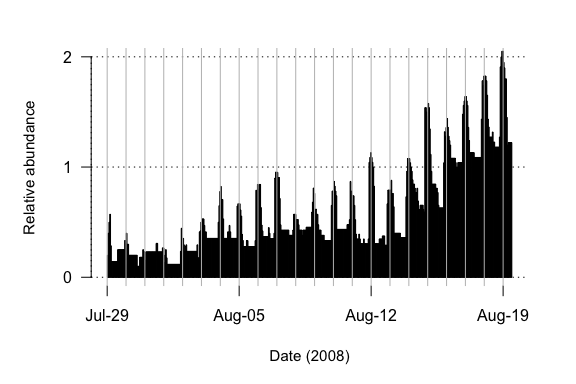# Example applications

#### 2021-04-19

The examples that follow use the datasets built into the linbin package to recreate the figures in the paper:

Ethan Z. Welty, Christian E. Torgersen, Samuel J. Brenkman, Jeffrey J. Duda, Jonathan B. Armstrong (2015). Multiscale analysis of river networks using the R package linbin. North American Journal of Fisheries Management, 35(4):802–809. doi: 10.1080/02755947.2015.1044764

### Elwha River : elwha

A survey of the Elwha River (Washington, USA) was conducted in August-September 2008 to collect physical variables and fish counts for hundreds of hydrologic units throughout the river mainstem. Due to high flow, several canyon sections were not safe to snorkel and therefore gaps are present in the data. In the example below, channel mean wetted width is plotted as a function of river distance for a number of different binning strategies — (a) original data flattened at overlaps only, (b) equal length bins (ignoring gaps), (c) equal coverage bins (straddling gaps), and (d) variable length bins (preserving gaps):

# Load event data
e <- elwha
e.filled <- fill_event_gaps(e, max.length = 1) # fill small gaps for the variable length bins (d)

# Design bins using different strategies
bins.a <- event_overlaps(e)[1:2]
bins.b <- seq_events(event_range(e), length.out = 33, adaptive = FALSE)
bins.c <- seq_events(event_coverage(e), length.out = 20, adaptive = FALSE)
bins.d <- seq_events(event_coverage(e.filled), length.out = 20, adaptive = TRUE)
bins <- rbind(cbind(bins.a, g = 1), cbind(bins.b, g = 2), cbind(bins.c, g = 3), cbind(bins.d, g = 4))

# Sample events at bins
e.bins <- sample_events(e, bins, list(weighted.mean, "mean.width", "unit.length"),
scaled.cols = "unit.length")

# Plot binned data
plot_events(e.bins, group.col = "g", data.cols = "mean.width", col = "grey", border = "#666666",
main = c("(a) Flattened original data", "(b) Equal length bins",
"(c) Equal coverage bins", "(d) Variable length bins"),
xlabs = "Distance upstream (km)", ylabs = "Wetted width (m)",
dim = c(4, 1), ylim = c(0, 56), xpd = NA)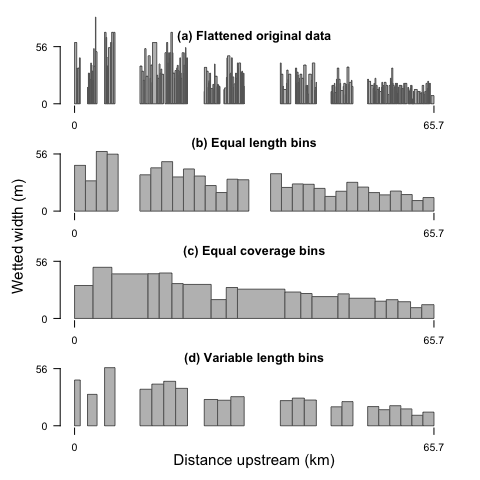### Quinault River : quinault

A survey of the Quinault River (Washington, USA) was conducted in August 2009 to collect physical variables and fish counts for hundreds of hydrologic units throughout the river mainstem. Total trout abundance (Oncorhynchus sp.) is plotted for a range of bin lengths:

# Load event data
e <- quinault

# Design bins
bin.lengths <- c(100, 200, 400, 800, 1600, 3200, 6400, 12800, 25600) # m
bins <- seq_events(event_range(e), by = bin.lengths / 1000) # km

# Sample events at bins
e.bins <- sample_events(e, bins, list(sum, "ONXX.*"), scaled.cols = "ONXX.*")

# Plot binned data
plot_events(e.bins, group.col = "group", data.cols = "ONXX.total",
main = paste0("Bin length = ", prettyNum(bin.lengths, ","), " m"),
xlabs = "Distance upstream (km)", ylabs = "Trout abundance",
dim = c(3, 3), byrow = TRUE, oma = c(3, 3, 2, 2))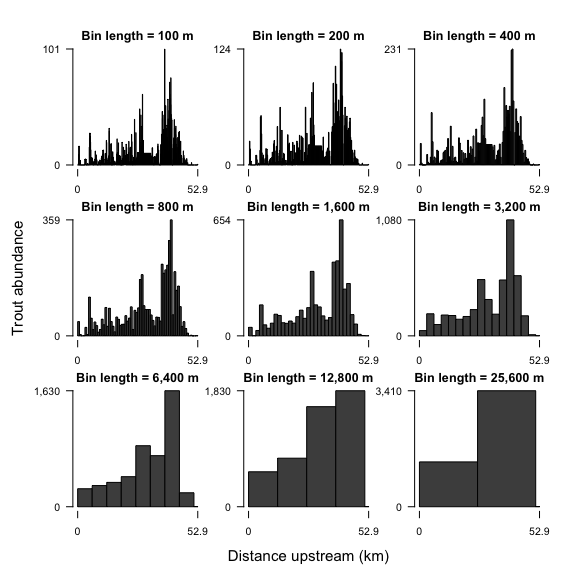Rather than plotting the total count, the individual size classes (10 - 20 cm, 20 - 30 cm, > 30 cm) are stacked together in the barplots:

plot_events(e.bins, group.col = "group", data.cols = "ONXX.[0-9]+",
main = paste0("Bin length = ", prettyNum(bin.lengths, ","), " m"),
xlabs = "Distance upstream (km)", ylabs = "Trout abundance",
dim = c(3, 3), byrow = TRUE, oma = c(3, 3, 2, 2), col = heat.colors(3), border = NA)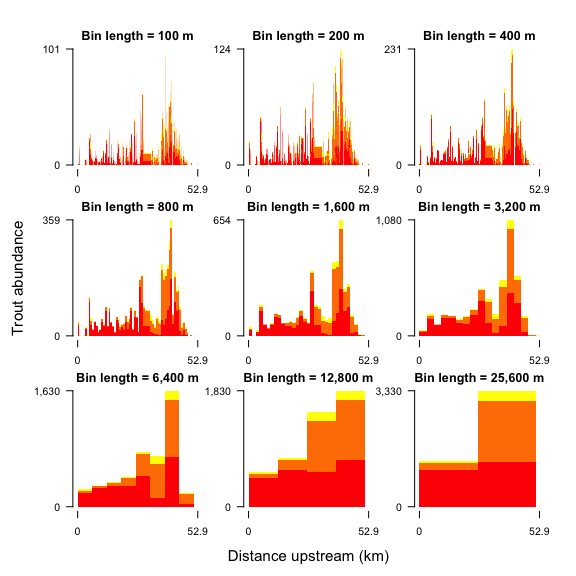### NetMap : netmap

NetMap (terrainworks.com) employs digital elevation models to generate detailed river networks and compute biophysical variables for spatially continuous hydrologic units throughout the networks. The included dataset contains standard NetMap output for the entire network of the Dungeness River (Washington, USA). In this example, NetMap variables are plotted by distance upstream from the river mouth both for the mainstem and for the entire network. The variables are binned as means weighted by stream length, and include intrinsic potential (IP, a modeled estimate of likelihood of occurrence) for Chinook Salmon (IP_CHINOOK), Coho Salmon (IP_COHO), and steelhead (IP_STEELHD), the fraction of favorable beaver habitat (BeavHab), and mean channel depth (DEPTH_M):

# Load NetMap data
d <- netmap

# Convert to event table
# (compute from and to endpoints from Netmap variables)
# (OUT_DIST = distance from outlet in km, LENGTH_M = length of unit in m)
d$from <- d$OUT_DIST
d$to <- d$from + (d$LENGTH_M / 1000) # Seperate into mainstem and network e.main <- d[d$CHAN_ID == 1, ]
e.net <- d

# Design bins
bins = seq_events(event_range(e.net), length.out = 10)

# Sample events at bins
fields = c("IP_CHINOOK", "IP_COHO", "IP_STEELHD", "BeavHab", "DEPTH_M")
e.bins.main = sample_events(e.main, bins, list(weighted.mean, fields, "LENGTH_M"),
scaled.cols = "LENGTH_M")
e.bins.net = sample_events(e.net, bins, list(weighted.mean, fields, "LENGTH_M"),
scaled.cols = "LENGTH_M")
e.bins = rbind(cbind(e.bins.main, group = 1), cbind(e.bins.net, group = 2))

# Plot binned data
plot_events(e.bins, group.col = 'group', data.cols = fields, sigfigs = c(3, 2),
xlabs = c('Distance upstream (km)\nmainstem', 'Distance upstream (km)\nnetwork'),
ylabs = c('Depth (m)', 'Proportion', 'IP', 'IP', 'IP'),
oma = c(4, 3, 2, 2), mar = c(2, 4, 1.5, 0.5))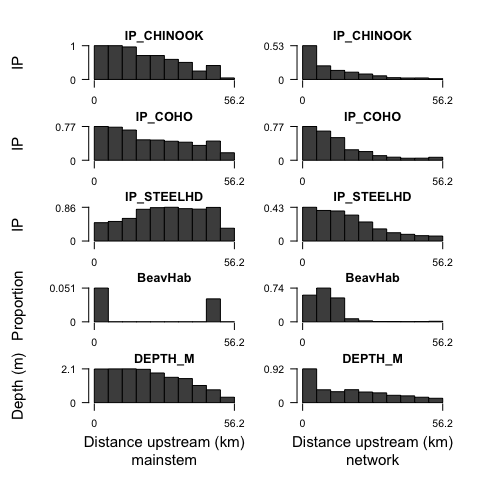### Fish Movements : fishmotion

This dataset contains a pair of event tables (in a list) documenting the movements of passive integrated transponder (PIT) tagged Coho Salmon (Oncorhynchus kisutch) in the downstream 1-km of Bear Creek (Southwest Alaska, USA) for 29 July - 19 August 2008. The first ($motion) lists individual fish residence time intervals in each of three stream regions, while the second ($origin) lists the study-wide residence time of each fish and the stream region in which the fish was first tagged. Event endpoints correspond to start and end times stored as seconds since 1970-01-01 UTC (POSIXct). The number of fish in region 1, normalized by the study-wide number of fish first tagged in region 1, is plotted below in 1-hour bins:

# Load event data
d = fishmotion
e.motion = d[]
e.origin = d[]

# Design hourly bins
# (endpoints are in seconds since 1970-01-01 UTC)
bins = seq_events(event_range(e.motion), by = 3600)

# Sample events at bins
e.motion.bins = sample_events(e.motion, bins, list(length, 'region', by = 'region'))
e.origin.bins = sample_events(e.origin, bins, list(length, 'region', by = 'region'))

# Normalize by total fish present tagged in region 1
e.motion.bins$fish.1.norm = e.motion.bins$region.1 / e.origin.bins$region.1 # Prepare weekly data labels bins[c("from.date", "to.date")] = lapply(bins[c("from", "to")], as.POSIXct, origin = '1970-01-01', tz = "US/Alaska") week.ticks = seq(trunc(min(bins$from.date), "day"), trunc(max(bins$from.date), "day"), by = "week") week.labels = format(week.ticks, '%b-%d') # Plot binned data plot_events(e.motion.bins, data.cols = "fish.1.norm", yticks = c(0, 1, 2), col = par("fg"), ylim = c(0, 2), plot.grid = TRUE, xpd = FALSE, main = NA, xlabs = "Date (2008)", ylabs = "Relative abundance", xticks = week.ticks, xtick.labels = week.labels, oma = c(3, 2, 1, 2)) # Add daily vertical lines days = seq(trunc(min(bins$from.date), "day"), trunc(max(bins\$from.date), "day"), by = "day")
abline(v = days, col = 'grey')